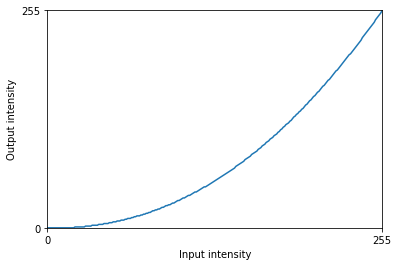Notebook

# Digital Image Processing

## Chapter 1: Point Operations

In :
%run load_lib_ch1.py


Point operations are functions performed on each pixel of an image, regardless of the other pixels. They can be unary or binary meaning that one or two images are used to produce the end result. Point operations can be visualized as graphs of the functions of the intensity at each pixel. The following graph shows the original image graph before any operation is performed.

In :
def no_operation(x):
return x

In :
image_function_graph(no_operation)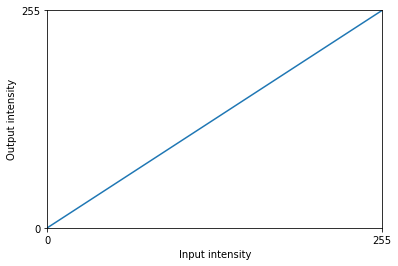A typical point operation applies a mathematical function on each pixel. The cell below defined a function $T(x,y) = f(x,y)/2$ which halves the intensity of each pixel. The resulting image appears as a darker version of the original.

In :
def fn_1(x):
x = np.asarray(x)
return (x/2).astype(np.uint8)

In :
compare_result(img, apply_point_op(img, fn_1))In :
image_function_graph(fn_1)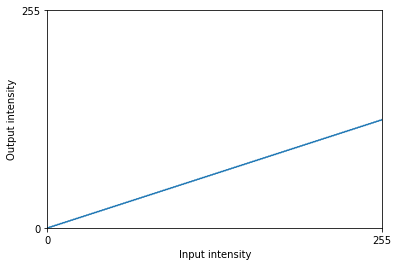Another typical example for a point operation is thresholding where each pixel is compared to a threshold and processed accordingly. Thresholding is often used to binarize an image to a black and white image, or to mask out details of a photo.

Bottom threshold:

$T(x,y) =$

$0$, for $f(x,y) < threshold$

$f(x,y)$, for $f(x,y) > threshold$

In :
def fn_thresh_bot (x):
thresh = 75
y = fn.rgb2luma(x)
if (y < thresh):
return [0,0,0]
else:
return x

In :
compare_result(img, apply_point_op(img, fn_thresh_bot))In :
image_function_graph(fn_thresh_bot)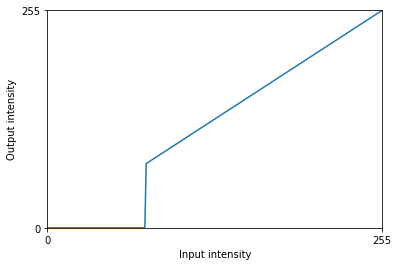Binarization:

$T(x,y) =$

$0$, for $f(x,y) < threshold$

$255$, for $f(x,y) > threshold$

In :
def fn_thresh_both (x):
thresh = 80
y = fn.rgb2luma(x)
if (y < thresh):
return [0,0,0]
else:
return [255, 255, 255]

In :
compare_result(img, apply_point_op(img, fn_thresh_both))A

In :
image_function_graph(fn_thresh_both)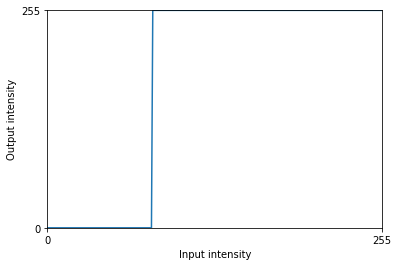Virtually any function of pixel intensity can be applied to produce a processed point operation.

### Gamma Correction

Gamma correction is a type of point operation that follows the formule $f(x,y) = c.r^\gamma$, where $c$ and $\gamma$ are the tuning parameters and $r$ is the normalized intensity. Gamma correction is widely used to correct the input radiance mapping of the camera pixels and display the image properly on monitors.

In :
def fn_gamma_correction (x):
x = np.asarray(x)
gamma = 2.2
r = 1
y = r*np.power(x/255.0,gamma)*255
return y.astype(np.uint8)

In :
image_function_graph(fn_gamma_correction)